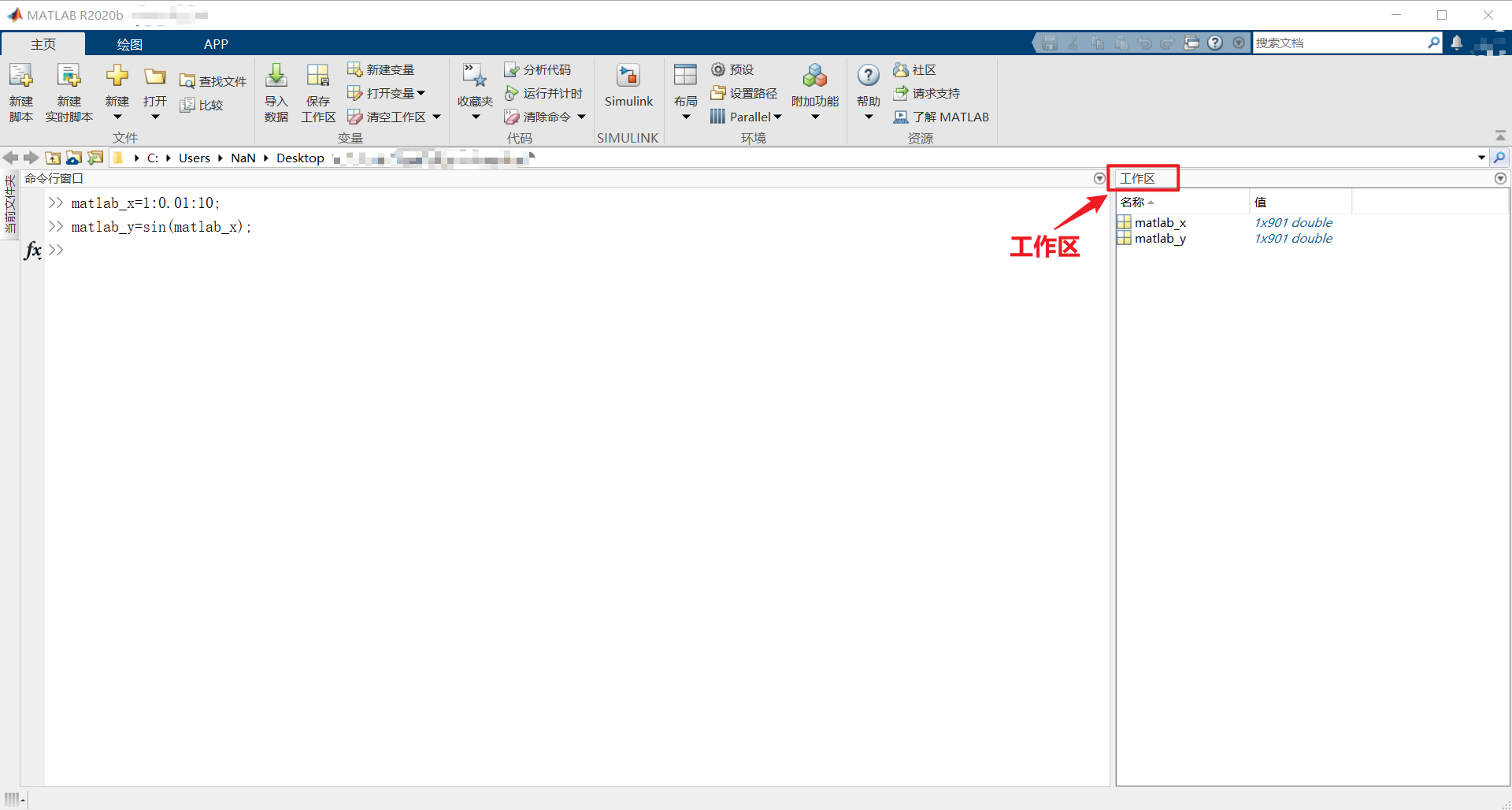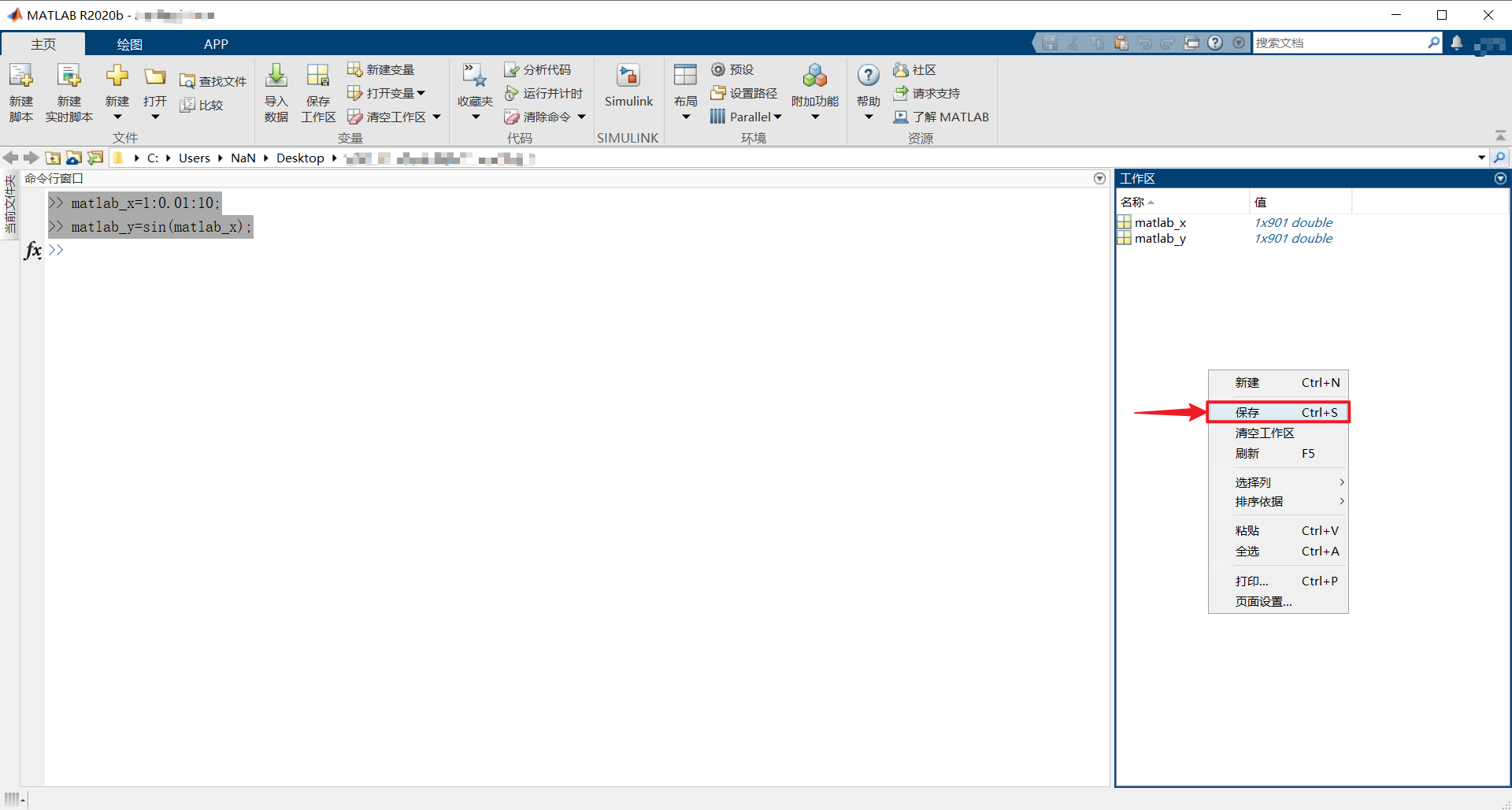# python学习笔记

+关注继续查看

﻿﻿@TOC

• 正数的补码等于原码
• 负数的补码等于符号位不变，其它位取反，再加1

+150b 0000 11110b 0000 1111
-150b 1000 00000b 1111 0001

• 符号位等于0(正数)，原码=补码import numpy as np
index = np.argwhere(int_number_array & 0b1000000000000000 == 0b1000000000000000)#查找数组中符号位为1(负数)，返回索引数组
int_number_array[index] = -((int_number_array[index] ^ 0b1111111111111111) + 1)#进行补码转换成原码(按位异或相当于按位取反)正数不变，负数进行取反加1，最后加负号
number_array = int_number_array / 32768 * 5 #将十六进制原码数据转换电压值
print(number_array)

[-1.52587891e-04 1.24984741e+00 6.22253418e-01 8.46557617e-01 8.44421387e-01 2.35671997e+00 1.52587891e-04 2.44140625e-03]

# python读取matlab数据(.mat文件)

1.首先，我们这里先打开matlab，随便在命令行窗输入两个变量，

matlab_x=1:0.01:10;
matlab_y=sin(matlab_x);

2.计算处理后，matlab右边的工作区会有两个变量值，分别为matlab_y、matlab_x3.然后，我们将鼠标放置在工作区空白位置右键，选择保存，也可以在工作区处于工作高亮状态时使用快捷键ctrl+s进行保存，会弹出保存文件名，这里我们保存为matlab.mat4.接下来就是用Python读取上一步中保存的matlab工作区的数据Data。Python中我们需要用到scipy库，这里我们先import进去

import scipy.io as scio

5.读取.mat文件

data=scio.loadmat('./matlab.mat')

6.查看当前data数据类型

type(data)

7.读取对应我们想要的数据

import numpy as np #导入矩阵处理库
python_y=np.array(data['matlab_y']) #将matlab数据赋值给python变量

enjoy it！Python 学习笔记 - 函数
892 0sed 学习笔记
905 0Python 学习笔记 - 装饰器
926 0Python 学习笔记 - 反射
920 0Hibernate 学习笔记
898 0Python 学习笔记 - 正则表达式模块
788 0Python学习的第一天
1005 0众所周知，Python语言简洁、功能强大，被看做是人工智能时代最佳的编程语言。然而golang语言的出现正在威胁Python的地位，试图取代Python成为机器学习以及AI的主流编程语言。有人好奇未来几年Python就业前景如何，如果想要从事Python开发又应该怎样高效学习Python呐?且看分析。
1549 01728 01595 0

You may create better art.
32

0

《SaaS模式云原生数据仓库应用场景实践》

《看见新力量：二》电子书